Nonequispaced fast Fourier transform
Fast Gauss transform
Fast Gauss transforms with complex parameters using NFFT

# Fast Gauss transforms with complex parameters using NFFT

This library of C functions computes approximations of sums of the following form. Given complex coefficients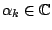and source knots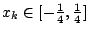, our goal consists in the fast evaluation of the sum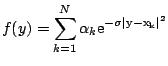at the target knots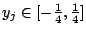,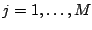, where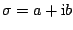,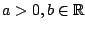denotes a complex parameter.

The algorithms are implemented by Stefan Kunis in ./applications/fastgauss. Related paper are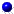Kunis, S., Potts, D. and Steidl, G.
Fast Gauss transforms with complex parameters using NFFTs.
J. Numer. Math. 14, 295-303.   (full paper ps, pdf),   2006